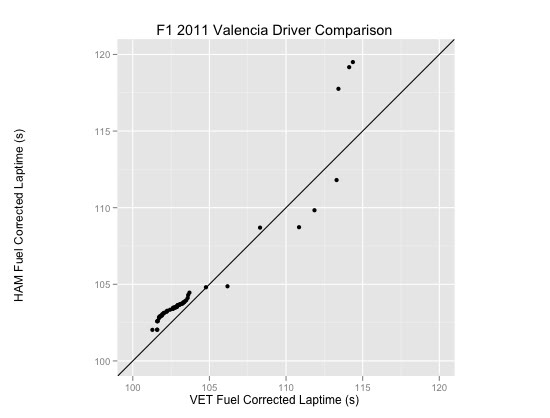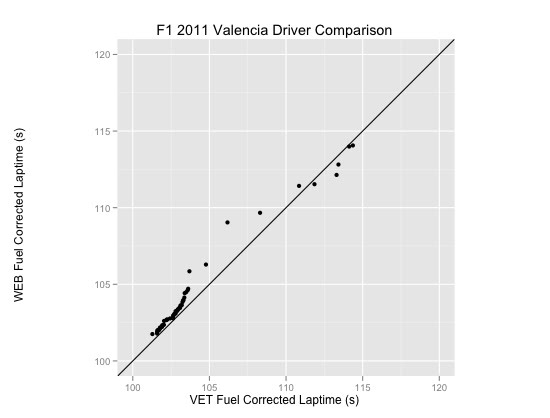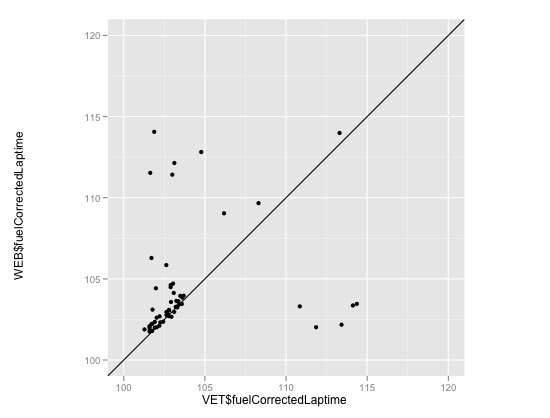## Monday, June 27, 2011

### F1 2011 European Grand Prix/Valencia - Race - More Driver Comparisons

**BEWARE-I messed up a config file and used Monaco settings for the fuel penalty calculations, so the fuel corrected laptime charts are currently meaningless:-( Thanks to @marussiavirgin for pointing it out**

Picking up on a couple of issues raised with the previous batch of driver comparison charts, such as the arbitrary aspect ratio, here's a quick recap of a Red Bull's driver comparison chart with a slightly neater presentation:Here's the R code for producing that image (with thanks to csgillespie for answering my question - CrossValidated/Stats StackExchange question 'How to compare two datasets with Q-Q plot using ggplot2?'):
HAM=subset(eur_2011comprehensiveLapTimes,car==3)
VET=subset(eur_2011comprehensiveLapTimes,car==1)
library(ggplot2)
sx=sort(VET\$fuelCorrectedLaptime)
sy=sort(HAM\$fuelCorrectedLaptime)
lenx <- length(sx)
leny <- length(sy)
if (leny < lenx)sx <- approx(1L:lenx, sx, n = leny)\$y if (leny > lenx)sy <- approx(1L:leny, sy, n = lenx)\$y g = ggplot() + geom_point(aes(x=sx, y=sy))+geom_abline(slope=1)+opts(aspect.ratio = 1)+xlim(100,120)+ylim(100,120)+labs(x='VET Fuel Corrected Laptime (s)',y='HAM Fuel Corrected Laptime (s)')+opts(title='F1 2011 Valencia Driver Comparison')

(I'm not sure how to shift the y-axis label closer to the actual axis?)

Here's another:(I wonder if these charts allow us to compare in/out laps (which lap incurs the actual pit stop time penalty? Those will be the really laps. The intermediate slow laps will be the other part of the pitstop phase, presumably?)

Unfortunately, I haven't been able to do the most trivial of things in qplot/ggplot - a scatterplot with aspect ratio.

PS Doh! All I had to do was crib the above code... here's a scatterplot (where we plot driver vs driver laptimes for the same lap, rather than in paired ranked order:Here's the code:
ggplot() + geom_point(aes(x=VET\$fuelCorrectedLaptime, y=WEB\$fuelCorrectedLaptime))+opts(aspect.ratio = 1)+geom_abline(slope=1)+xlim(100,120)+ylim(100,120)